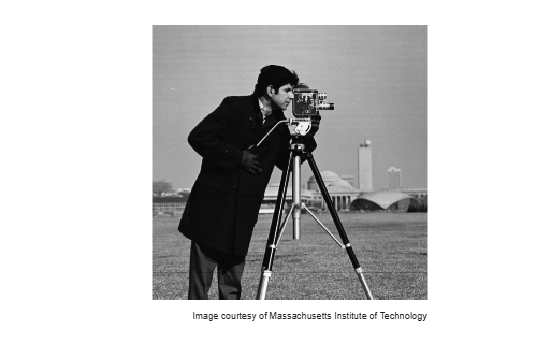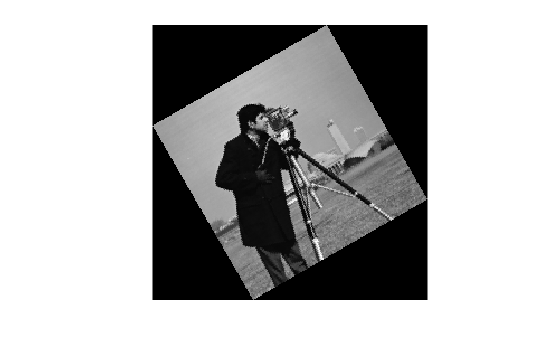Main Content

# Find Image Rotation and Scale

This example shows how to align or register two images that differ by a rotation and a scale change. You can use `fitgeotrans` to find the rotation angle and scale factor after manually picking corresponding points. You can then transform the distorted image to recover the original image.

### Step 1: Read Image

Read an image into the workspace.

```original = imread('cameraman.tif'); imshow(original) text(size(original,2),size(original,1)+15, ... 'Image courtesy of Massachusetts Institute of Technology', ... 'FontSize',7,'HorizontalAlignment','right')```### Step 2: Resize and Rotate the Image

```scale = 0.7; distorted = imresize(original,scale); % Try varying the scale factor. theta = 30; distorted = imrotate(distorted,theta); % Try varying the angle, theta. imshow(distorted)```### Step 3: Select Control Points

Use the Control Point Selection Tool to pick at least two pairs of control points.

```movingPoints = [151.52 164.79; 131.40 79.04]; fixedPoints = [135.26 200.15; 170.30 79.30];```

You can run the rest of the example with these pre-picked points, but try picking your own points to see how the results vary.

`cpselect(distorted,original,movingPoints,fixedPoints);`

Save control points by choosing the File menu, then the Save Points to Workspace option. Save the points, overwriting variables `movingPoints` and `fixedPoints`.

### Step 4: Estimate Transformation

Fit a nonreflective similarity transformation to your control points.

`tform = fitgeotrans(movingPoints,fixedPoints,'nonreflectivesimilarity');`

After you have done Steps 5 and 6, repeat Steps 4 through 6 but try using 'affine' instead of 'NonreflectiveSimilarity'. What happens? Are the results as good as they were with 'NonreflectiveSimilarity'?

### Step 5: Solve for Scale and Angle

The geometric transformation, `tform`, contains a transformation matrix in `tform.T`. Since you know that the transformation includes only rotation and scaling, the math is relatively simple to recover the scale and angle.

Let $\mathrm{sc}=\mathrm{scale}*\mathrm{cos}\left(\mathrm{theta}\right)$

Let $\mathrm{ss}\text{\hspace{0.17em}}=\text{\hspace{0.17em}}\mathrm{scale}*\mathrm{sin}\left(\mathrm{theta}\right)$

Then, `Tinv = invert(tform)`, and `Tinv.T = `$\left[\begin{array}{ccc}\mathrm{sc}& -\mathrm{ss}& 0\\ \mathrm{ss}& \mathrm{sc}& 0\\ \mathrm{tx}& \mathrm{ty}& 1\end{array}\right]$

where `tx` and `ty` are x and y translations, respectively.

```tformInv = invert(tform); Tinv = tformInv.T; ss = Tinv(2,1); sc = Tinv(1,1); scale_recovered = sqrt(ss*ss + sc*sc)```
```scale_recovered = 0.7000 ```
`theta_recovered = atan2(ss,sc)*180/pi`
```theta_recovered = 29.3741 ```

The recovered values of `scale_recovered` and `theta_recovered` should match the values you set in Step 2: Resize and Rotate the Image.

### Step 6: Recover Original Image

Recover the original image by transforming `distorted`, the rotated-and-scaled image, using the geometric transformation `tform` and what you know about the spatial referencing of `original`. The 'OutputView' name-value pair is used to specify the resolution and grid size of the resampled output image.

```Roriginal = imref2d(size(original)); recovered = imwarp(distorted,tform,'OutputView',Roriginal);```

Compare `recovered` to `original` by looking at them side-by-side in a montage.

`montage({original,recovered})`The `recovered` (right) image quality does not match the `original` (left) image because of the distortion and recovery process. In particular, the image shrinking causes loss of information. The artifacts around the edges are due to the limited accuracy of the transformation. If you were to pick more points in Step 3: Select Control Points, the transformation would be more accurate.

Download ebook